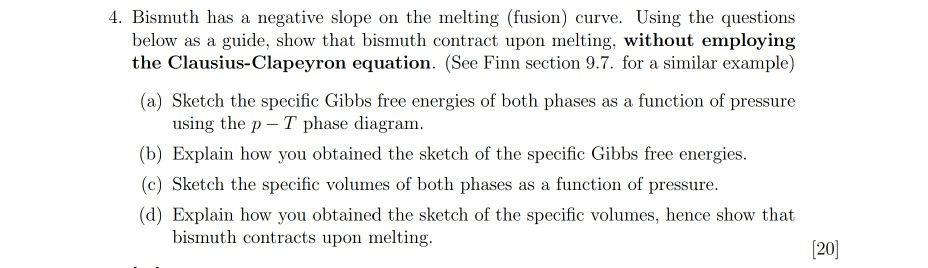# Question 4. Bismuth has a negative slope on the melting (fusion) curve. Using the questions below as a guide, show that bismuth contract upon melting, without employing the Clausius-Clapeyron equation. (See Finn section 9.7. for a similar example) (a) Sketch the specific Gibbs free energies of both phases as a function of pressure using the p-T phase diagram. (b) Explain how you obtained the sketch of the specific Gibbs free energies. (c) Sketch the specific volumes of both phases as a function of pressure. (d) Explain how you obtained the sketch of the specific volumes, hence show that bismuth contracts upon melting.ZALNLT The Asker · Chemical EngineeringTranscribed Image Text: 4. Bismuth has a negative slope on the melting (fusion) curve. Using the questions below as a guide, show that bismuth contract upon melting, without employing the Clausius-Clapeyron equation. (See Finn section 9.7. for a similar example) (a) Sketch the specific Gibbs free energies of both phases as a function of pressure using the p-T phase diagram. (b) Explain how you obtained the sketch of the specific Gibbs free energies. (c) Sketch the specific volumes of both phases as a function of pressure. (d) Explain how you obtained the sketch of the specific volumes, hence show that bismuth contracts upon melting. 
More
Transcribed Image Text: 4. Bismuth has a negative slope on the melting (fusion) curve. Using the questions below as a guide, show that bismuth contract upon melting, without employing the Clausius-Clapeyron equation. (See Finn section 9.7. for a similar example) (a) Sketch the specific Gibbs free energies of both phases as a function of pressure using the p-T phase diagram. (b) Explain how you obtained the sketch of the specific Gibbs free energies. (c) Sketch the specific volumes of both phases as a function of pressure. (d) Explain how you obtained the sketch of the specific volumes, hence show that bismuth contracts upon melting.